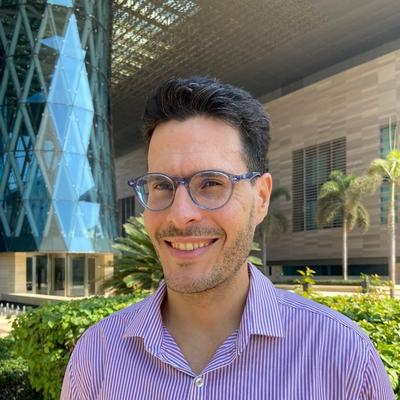Retour aux activités
Séminaire informel de théorie des systèmes (ISS)

# MFG price models with common noise

iCalendar

### Ricardo D. Ribeiro – King Abdullah University of Science and Technology, Arabie saouditeLien pour le webinaire
ID de réunion : 910 7928 6959
Code secret : VISS

The problem of determining a just price arises in market economies, including electricity generation from renewable sources in smart grids. In this talk, we will review the deterministic MFG price formation model (Gomes-Saúde). Then we generalize it to an MFG model for the price formation of a commodity whose production is subject to random fluctuations. Here, agents seek to minimize their average cost by choosing their trading rates with a price characterized by a balance between supply and demand. The supply and the price processes are assumed to follow stochastic differential equations. We show that the optimal trading rates are determined in a feedback form for linear dynamics and quadratic costs. Hence, the price appears as the solution to a stochastic differential equation, whose coefficients depend on a system of ordinary differential equations.Then we consider a discredited version; a finite number of players trade an asset whose supply is a stochastic process. By solving a constrained minimization problem, we prove that the Lagrange multiplier corresponding to the market-clearing condition defines the solution of the price formation problem. For the linear-quadratic structure, we characterize the price process using optimal control techniques. We include two numerical approaches for the price computation.

Biography: Ricardo does research in MFGs and PDEs at the Computer, Electrical and Mathematical Sciences and Engineering at the King Abdullah University of Science and Technology (CEMSE-KAUST) since 2019. Before that he was Professor of Mathematics at UNICAMP (1 year) and UTFPR-Londrina (4 years). He did his PhD under supervision of professors Manuel Valentim Garcia (advisor) and Diogo A. Gomes (co-advisor).Peter E. Caines responsable